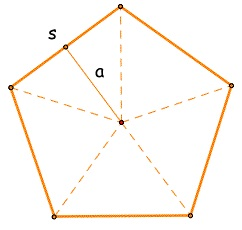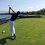# Proof for area of any regular polygonThis note is a follow up for my circle area proof here

In this note I will prove that the area of any regular polygon can be written as $\frac{ap}{2}$ where a is the anothem and p is the perimeter. I will try my best to make this understandable but it is much easier to understand this proof through video.

In the picture above, we see that all regular polygons can be divided into congruent triangles. The area of a triangle is of course bh/2. However, the triangle's height is equal to the apothem of the original polygon. Thus we have a(b/2).

Now, if we look at all the bases of the triangles, they add up to the perimeter of the original polygon. So the total area of the original polygon is the total area of all the triangles which is $a\frac{bn}{2}$ where n is the number of sides.

Finally, since bn= the perimeter of the polygon, we arrive at the conclusion that $\frac{ap}{2}$ is the area of the original polygon.

Thank you for the challenge @JubayerNirjhor: In my next note, I will prove that the area of any regular polygon can be represented as

$\dfrac{1}{4} na^2\cot\dfrac{\pi}{n}$. Where n is the number of sides and a is the side length. You can find it hereNote by Trevor Arashiro
6 years, 10 months ago

This discussion board is a place to discuss our Daily Challenges and the math and science related to those challenges. Explanations are more than just a solution — they should explain the steps and thinking strategies that you used to obtain the solution. Comments should further the discussion of math and science.

When posting on Brilliant:

• Use the emojis to react to an explanation, whether you're congratulating a job well done , or just really confused .
• Ask specific questions about the challenge or the steps in somebody's explanation. Well-posed questions can add a lot to the discussion, but posting "I don't understand!" doesn't help anyone.
• Try to contribute something new to the discussion, whether it is an extension, generalization or other idea related to the challenge.

MarkdownAppears as
*italics* or _italics_ italics
**bold** or __bold__ bold
- bulleted- list
• bulleted
• list
1. numbered2. list
1. numbered
2. list
Note: you must add a full line of space before and after lists for them to show up correctly
paragraph 1paragraph 2

paragraph 1

paragraph 2

[example link](https://brilliant.org)example link
> This is a quote
This is a quote
    # I indented these lines
# 4 spaces, and now they show
# up as a code block.

print "hello world"
# I indented these lines
# 4 spaces, and now they show
# up as a code block.

print "hello world"
MathAppears as
Remember to wrap math in $$ ... $$ or $ ... $ to ensure proper formatting.
2 \times 3 $2 \times 3$
2^{34} $2^{34}$
a_{i-1} $a_{i-1}$
\frac{2}{3} $\frac{2}{3}$
\sqrt{2} $\sqrt{2}$
\sum_{i=1}^3 $\sum_{i=1}^3$
\sin \theta $\sin \theta$
\boxed{123} $\boxed{123}$

Sort by:

In most circumstances, people don't know the length of the apothem, but the length of a side. So it's useful to have an area formula with side length and number of sides argument.

If $n$ is the number of sides and $a$ is the length of one side, then the formula is $\dfrac{1}{4} na^2\cot\dfrac{\pi}{n}$. (Prove it)

- 6 years, 10 months ago

I will try. But trig is not my strong suit.

- 6 years, 10 months ago

Got it! It was a fun proof to do, took me about 10 mins because I was thinking of it the wrong way (trying to use identities). I'll post the proof in just a sec.

- 6 years, 10 months ago

@Trevor Arashiro Can you add this discussion (along with part 2) to the Regular Polygons - Area wiki page? Thanks!

Staff - 6 years, 9 months ago